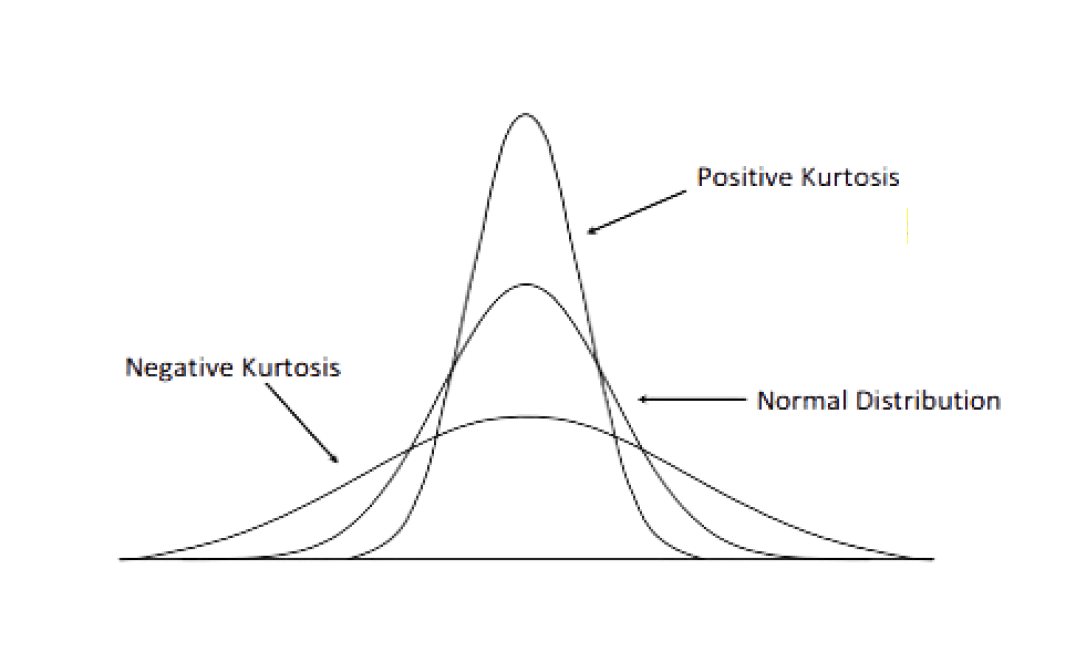## Kurtosis

Sample kurtosis is a measure of the “tailedness” of the probability distribution. Kurtosis is how much the shape of a distribution differs from a normal curve in terms of whether its curve in the middle is more peaked or flat than the normal curve. The kurtosis of the standard normal distribution is 3.

Distributions with kurtosis less than 3 (negative kurtosis) have a flatter curve with fewer scores in the tails than the normal distributions and are said to be thin-tailed. An example of a thin-tailed distribution is the uniform distribution, which does not produce outliers.

Distributions with kurtosis more than 3 (positive kurtosis) have a very peaked curve with more scores in the tails than the normal distribution. They are said to be heavy-tailed or fat tailed. An example of a fat-tailed distribution is the Laplace distribution, which has tails that asymptotically approach zero more slowly than a Gaussian, and therefore produces more outliers than the normal distribution.Often, we use excess kurtosis, which is defined as kurtosis k minus 3, to provide comparison to the normal distribution. It is defined as$g_{2}=k-3=\frac{\frac{1}{n}\sum_{i=1}^{n}{(x_{i}-\bar{x})^{4}}}{(\frac{1}{n-1}\sum_{i=1}^{n}{(x_{i}-\bar{x})^{2}})^{2}}-3=\frac{\frac{1}{n}\sum_{i=1}^{n}{(x_{i}-\bar{x})^{4}}}{s^{4}}-3$

In 1986. Moors gave an interpretation of kurtosis in the form of z-score (also known as standard score). It is the number of standard deviations a data point is above or below the mean value. Scores above the mean will have positive values, while those below the mean have negative values. Z-score is often a way to compare random variables of different distributions so they have the “same” unit. Z-score is defined as$Z=\frac{X-\mu}{\sigma}$

Combining the kurtosis and z-score,$k=E(Z^{4})=var(Z^{2})+[E(Z^{2})]^{2}$$=var(Z^{2})+(var(Z))^{2}$    (since$E(Z^{2})=var(Z)+(E(Z))^{2}$ and$E(Z)=0$ by definition)$var(Z^{2})+1$    (since$var(Z)=1$ by definition)

Kurtosis can therefore be seen as a measure of the dispersion of$Z^{2}$ around its expectation, or simply the dispersion of$Z$ around +1 and -1. For the original variable$X$, the kurtosis is a measure of the dispersion of$X$ around$\mu\pm\sigma$.$k$ attains its minimum value of 1 in a symmetrically two-point distribution. High values of kurtosis occurs in two situations:

1. The probability mass is concentrated around the mean. (peaked unimodal distribution)
2. The probability mass is concentrated in the tails of the distribution.

Example

For the sample$X=\{1,1,2,1,2,3,2,1,0\}$, the sample kurtosis is$g_{2}=\frac{\frac{1}{9}\sum_{i=1}^{9}{(x_{i}-1.4444)^{4}}}{(\frac{1}{8}\sum_{i=1}^{9}{(x_{i}-1.4444)^{2}})^{2}}-3=-1.04384$

Code

In NM Dev, we can use the class Kurtosis to compute the kurtosis of a data set using the above formula.

				
// create an array of doubles for our dataset
val values = doubleArrayOf(1.0, 1.0, 2.0, 1.0, 2.0, 3.0, 2.0, 1.0, 0.0)

// create the Kurtosis object
val kurtosis = Kurtosis(values)

println("Sample kurtosis: " + kurtosis.value())


				
Sample kurtosis: -1.043839758125472



## Thinking Time

What is kurtosis used for?

It is a useful measure of whether there is a problem with outliers in a data set. Larger kurtosis indicates a more serious outlier problem and may lead the researcher to choose alternative statistical methods.